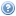# Course List for 2019

<- 2018The undergraduate courses on this list are also available in a timetable view.

Course Title Trimester(s) Teaching Home
COMP 312 Simulation and Stochastic Models 1
ENGR 123 Engineering Mathematics with Logic and Statistics 2, 3
MATH 277 Mathematical Statistics 1
MATH 309 Mathematical Logic 2
MATH 311 Algebra 1
MATH 318 Hilbert Spaces 2
MATH 335 Computability and Complexity 1
MATH 377 Probability and Random Processes 1
MATH 433 Model Theory 2
MATH 434 Set Theory 1
MATH 435 Computability and Complexity 2
MATH 436 Galois Theory and Number Theory 1
MATH 441 Measure Theory 1
MATH 461 Differential Equations 2
MATH 477 Probability 1
MATH 482 Special Topic: Topics in Machine Learning 2
MATH 483 Special Topic: Operator Algebra 2
STAT 193 Statistics in Practice 1, 2, 3
STAT 332 Statistical Inference 2
STAT 393 Linear Models 2
STAT 432 Computational Statistics 2
STAT 433 Stochastic Processes 2
STAT 434 Statistical Inference 2